Fall 2003

# Mathematics Math21b Fall 2003

## Linear Algebra and Differential Equations

Office: SciCtr 434
Email: knill@math.harvard.edu# The hydrogen atom

(An eigenvalue problem)
The linear operator
 ```T(f) = -fxx-fyy-fzz-2/r f ```
on smooth functions in R3 has eigenfunctions fn,l,m to the eigenvalues -n-2. The eigenfunctions are of the form
 ```fn,l,m = Rn,l(r) Yl,m(t,s) ```
with so called spherical harmonics Yl,m(t,s)=Pl|m|(cos(t)) exp(i m s). The functions Plm(z) are called Legendre spherical functions and Rn,l(r) are called Laguerre polynomials. T is the energy operator of the hydrogen atom. For each n, there are n2 eigenfunctions. The function f describes an electron with energy -n-2. The energy differences 1/n12 - 1/n22 can literally be "seen". The number n is called the principal quantum number, the number l is related to the total angular momentum, and m is related to the z-component of the angular momentum.
Lyman Balmer Paschen Bracket
n1=1 n1=2 n1=3 n1=4
Ultraviolet visible infrared infrared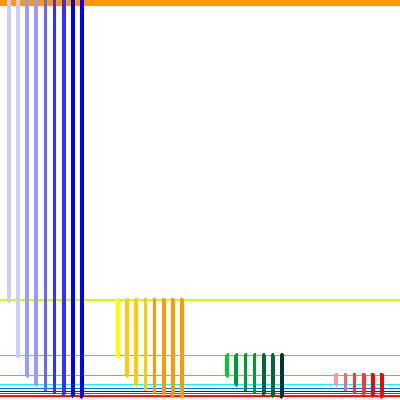## Visualization of the Spherical Harmonics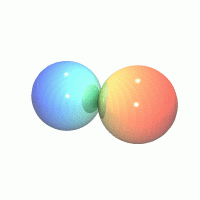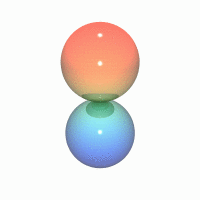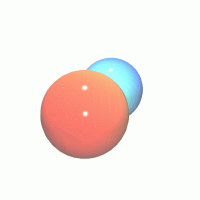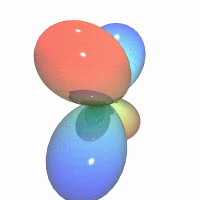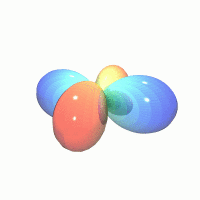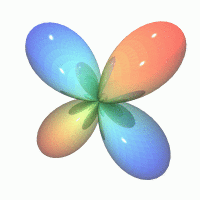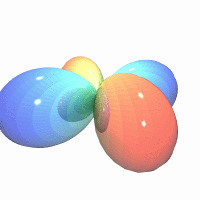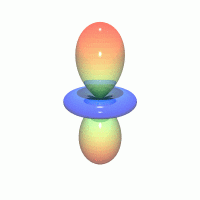## Visualization of the Laguerre polynomials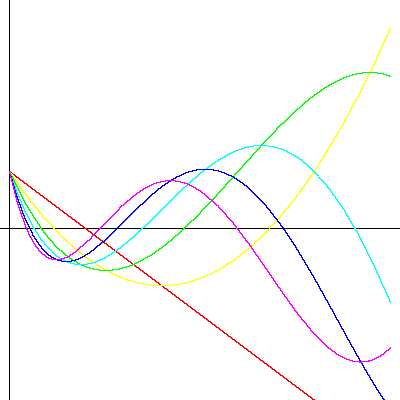Back to the main page